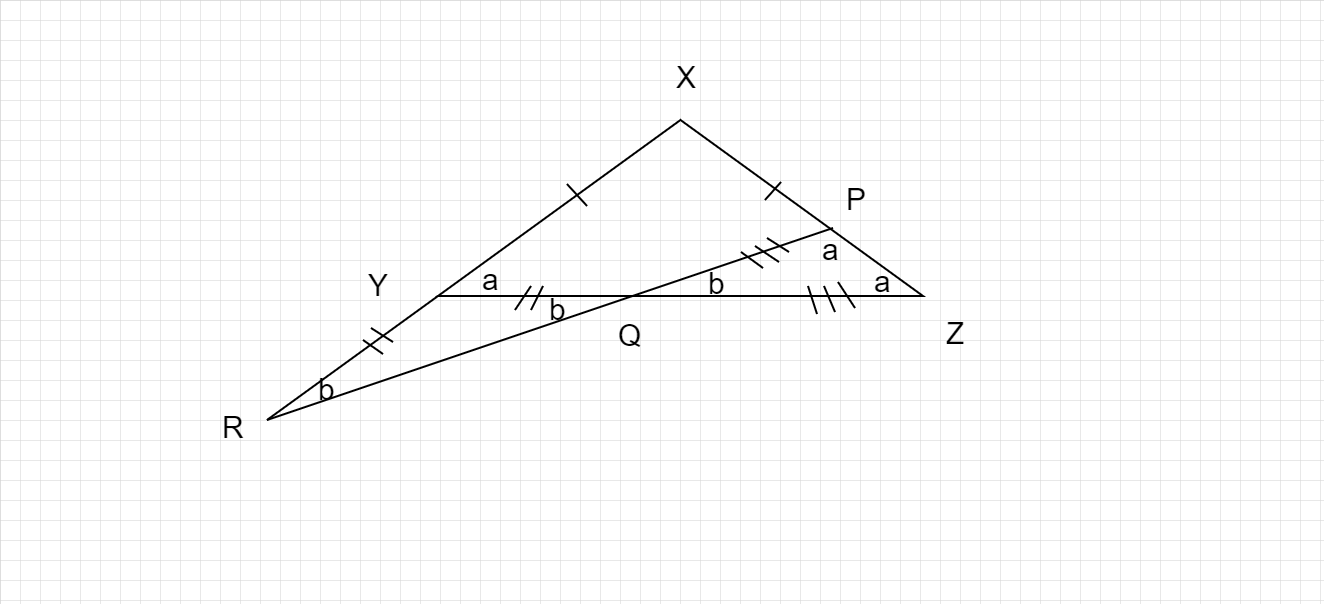# In $\Delta X Y Z, X Y=X Z$. A straight line cuts $X Z$ at $P, Y Z$ at $Q$ and $X Y$ produced at $R$. If $Y Q=Y R$ and $Q P=Q Z$, find the angles of $\Delta X Y Z$.

Given :

In Triangle XYZ ,

XY = XZ , YQ = YR  ;  QP = QZ.

To Find :

$$\displaystyle \angle XYZ\ ,\ \angle XZY\ ,\ \angle YXZ\ \$$

Solution :In triangle XYZ ,

XY  =  XZ

So, let's take

$\displaystyle \ \angle XYZ\ =\ \angle XZY\ =\ a$

In triangle YRQ ,

YQ  =  YR

So, let's take

$\displaystyle \ \angle YQR\ =\ \angle YRQ\ =\ b$

In triangle PQZ ,

PQ  =  QZ

So, let's take

$\displaystyle \ \angle PZQ\ =\ \angle QPZ\ =\ a$

For triangle YRQ, 'a' is Exterior angle, and 'b' , 'b' are interior angles.

By Exterior angle property,

Sum of two angles is equal to Exterior angle opposite to them.

$a=b+b$

$a=2b$............................... ( i)

$∠YQR =∠PQZ=b$                 (Vertically opposite angles)

In triangle PQZ,

Sum of all angles of triangle is equal to 180°

$a+a+b=180°$

$2a+b=180°$ ...............................( ii)

Substitute (i)  in  (ii)

$2a+b=180°$

$2(2b)+b=180°$

$4b+b=180°$

$5b=180°$

$b=\frac{180°}{5}$

$b=36°$

Substitute b = 36° in (i)

$a=2b$

$a=2\times36°$

$a=72°$

$∠XYZ\ =∠XZY=a$

So,

∠XYZ = 72° and

∠XZY = 72°

$\angle XYZ\ \ +\ \angle XZY\ +\ \angle YZX\ =\ 180°$

72° +72° + ∠YZX = 180°

144° + ∠YZX =180°

∠YZX = 180° $-$144°

∠YZX = 36°

So, the angles of triangle XYZ are,

$\angle XYZ =72°$

$\angle XZY =72°$

$\angle YZX =36°$.

Updated on: 10-Oct-2022

69 Views